Contents[Hide]

## 1. Overview

In Dundas BI, you can apply formulas to data by writing small expressions, much like you would in a spreadsheet program. A formula expression can be a simple constant value, arithmetic or other math functions, a call to a built-in formula function such as AVG or TOP, or any combination of these. For more complex formulas, you can write DundasScript.

Although this article demonstrates the various ways of using formulas while editing a dashboard or another view, you can add formula measures when editing a metric set full-screen as well.

Related video: Formulas

## 2. Accessing the formula bar

Select a data visualization on the canvas, click Data Tools in the toolbar and then Add Formula. (When editing a metric set full-screen, simply choose Add Formula in the toolbar).

A formula bar appears below the main toolbar.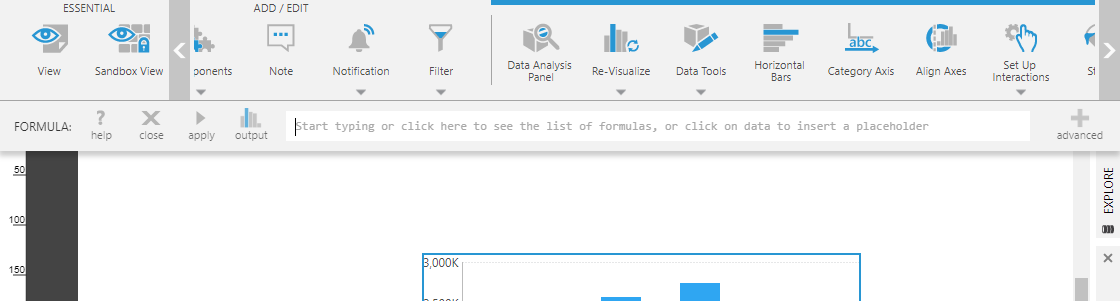Formula bar appears below the main toolbar

There is an output option for charts, for example, to choose what chart type to use to visualize the new formula measure. There is also an advanced mode for entering complex, multi-line formula expressions.

Once you are finished entering your formula, click apply or type Enter.

An alternative way of opening the formula bar is from the Data Analysis Panel: click to add a measure under Measures.

Then click <Formula> at the bottom under Calculated Elements.

## 3. Simple formulas

By default, the formula bar starts off in simple editing mode which allows you to enter a one line expression.

### 3.1. Constant value

The simplest formula expression you can have is a constant number. As an example, consider a bar chart showing order quantity as a measure by invoice date.

Open the formula bar for this chart and click the output button to change it from a bar chart series to another type.

Select the Line chart option and then click Submit.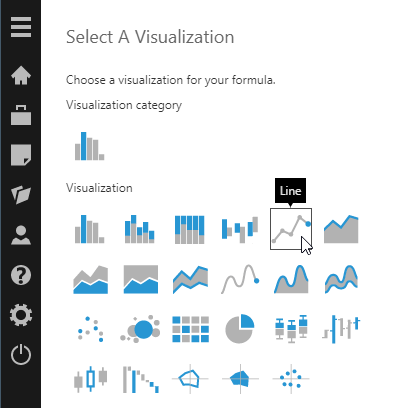Choose the type of visualization for the formula result

Notice that the icon for the output button changes to indicate a Line chart visualization.

Type a number into the formula bar and click apply or type Enter.

The text box is highlighted in blue and a checkmark appears on the right to indicate the formula syntax is correct, and the formula result is added as a line series.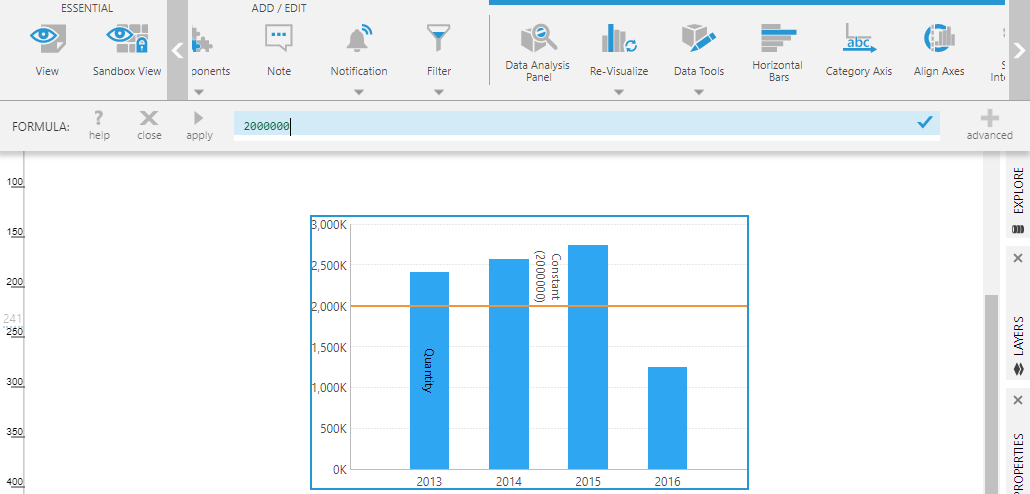A constant value line series is added to the chart

Open the Data Analysis Panel for the chart to see the formula result has been added as a second measure to the underlying metric set, appearing with an fx icon.

### 3.2. Placeholders

A formula expression can include placeholders representing the other data in your metric set. To insert a placeholder, type a dollar sign (\$) character in the formula bar. You will see a menu that lists available placeholders you can insert.

The inserted placeholder consists of the measure or hierarchy name enclosed between dollar sign characters (for example, \$OrderQty\$).

Another way to insert a placeholder is to click the relevant part of a data visualization. For example, click a column in a table, click a data point in a chart to insert a placeholder for the corresponding measure, or click an axis label.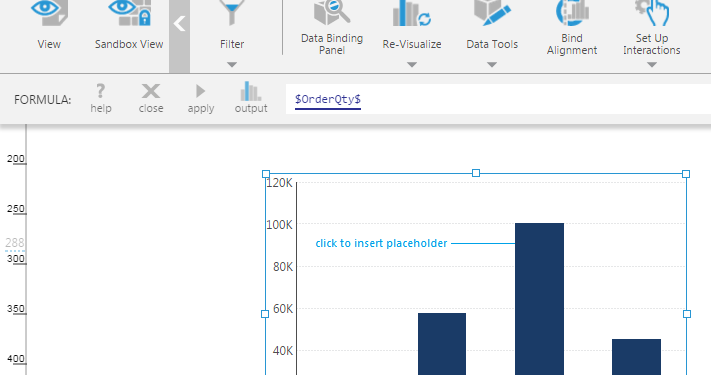Insert a placeholder by clicking on a data point

If you are working directly with hierarchy values in a formula, you can type a period (.) and one of the properties MemberTimeMemberNumberUniqueName, or Caption, or choose it from the popup. (If no property is specified, Caption is used by default.)

Formulas are measures so they should return numeric values. Some examples of referring to hierarchy values are \$ProductID\$.MemberNumber if the hierarchy contains numeric values, or for text values:

`if (\$HierarchyMember\$.Caption == "Test") { return 1; }`

#### 3.2.1. Placeholder settings

For some additional options, after adding a measure placeholder to your formula, click it to open the Placeholder dialog.

(When first adding the placeholder, click apply before clicking on the placeholder.)

To refer to values from other rows or columns, set Offset Value and Offset Axis. For example, an offset of -1 Rows can refer to the previous row's value.

The Aggregator can optionally be set to get the overall aggregated sum, average, etc., just for the values inserted by this placeholder into the formula.

### 3.3. Math

You can use arithmetic operators within a formula expression as well as math functions such as Math.Max() and Math.Abs(). These arithmetic operations and math functions are calculated separately in each row.

Examples:

• \$OrderQty\$ * 0.5
• Math.Abs(-0.75 * \$OrderQty\$)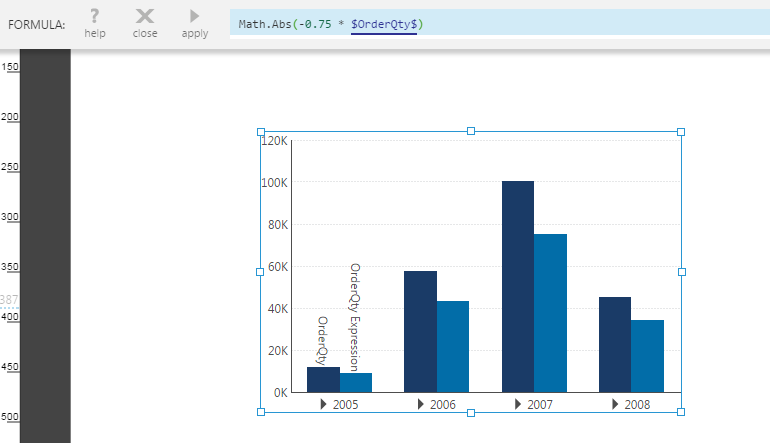Use a Math method like Math.Abs()

### 3.4. Functions

Dundas BI includes over 50 built-in formula functions that you can apply to your data. These functions work with the entire series of values you specify when calculating their result.

Type a letter in the formula bar and you'll see an auto-complete menu showing a list of matching functions you can choose from.

Once you've chosen a function (or typed it in manually along with the open bracket), a tooltip appears which shows the required and optional arguments for the function.

The first argument for the AVG function is a measure placeholder which you can insert by typing a dollar sign character or clicking on a data point.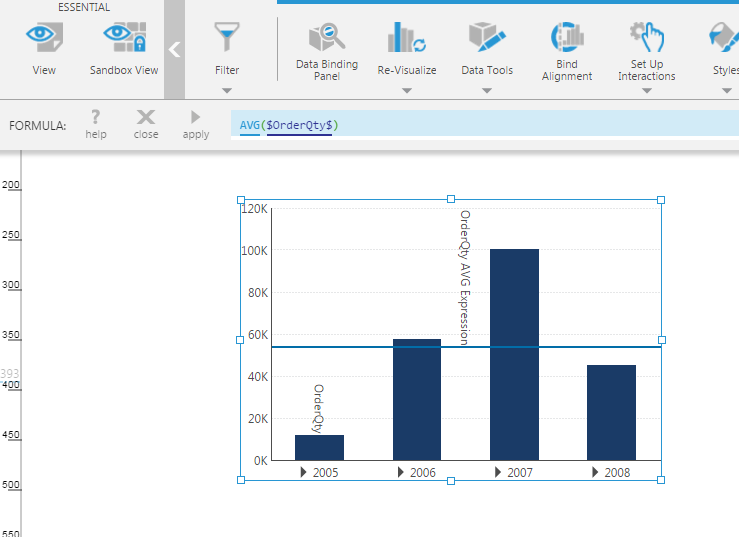Apply the AVG function to OrderQty

## 4. Advanced mode

Click the advanced button on the right of the formula bar to go into advanced editing mode. This button works like a toggle allowing you to flip between simple and advanced modes.

In advanced mode, you can enter multiple lines of script to more easily include if statements and other syntax available when using DundasScript. The formula should return a value using the return statement for advanced mode, even if it is just a single line.

For example, a constant formula must be entered as:

`return 40000;`

Tip
You can also write if/else statements on a single line in simple mode. For example:
if (\$Measure\$ > 100) return 1; else if (\$Measure\$ < 0) return -1; else return 0;

## 5. Formula visualizations

When editing a dashboard or another view, click Data Visualization, and then click Formula Visualization under the Formula category.

This opens the formula bar just like for adding a formula measure, except that you are able to optionally refer to data from multiple visualizations or metric sets rather than just one.

When you click apply, an entirely new metric set is created and displayed in a new visualization instead of modifying an existing visualization and metric set. This new metric set is called a formula metric set, and it is able to produce an entirely different output than the metric set(s) it was based on, potentially consisting of different rows or columns as well as different measure values.

For a walkthrough on adding formula visualizations, see Using a formula visualization.

## 6. Editing a formula

Once you've added a formula measure, you can go back and edit it by clicking the fx button in the Data Analysis Panel.

The formula bar opens and the corresponding formula is loaded into the formula bar.

You can make changes to the formula and then click apply.

## 7. Parameter placeholders

In addition to placeholders for measures and hierarchies, formulas support parameter placeholders, which allow you to refer to the value of a parameter connected to a filter or set in script. This can allow viewers of dashboards and other views to enter their own value into the formula, pass in a value through an interaction, or for custom functionality implemented in script to affect the formula when viewing.

To create a parameter placeholder, simply type one in when adding or editing any formula. Choose a name that's not already taken by an element in your metric set, and surround it with dollar signs (\$):

(After typing a dollar sign, a popup menu will list the names that are already taken).

The placeholder can either appear by itself, or be used anywhere in the formula you would like to be able to change the value later. Click apply when done.

This creates a parameter that is numeric. You may want a parameter that isn't numeric, especially if using the advanced mode of the formula bar to write script into your formula. You can change its type by clicking on the placeholder you just typed, which is now underlined, then clicking Parameter placeholder properties....

Go to Filters in the toolbar and add a filter. Or, to set the parameter value through an interaction or through script, you can open the Parameters window and add a new view parameter.

In the connections list that appears, un-check any existing connections, which are for regular filter parameters, and then find and expand your formula measure. Connect to the (Single Number) parameter under the formula measure. (If you changed the type of the parameter, look for the single value of that type instead).

For a numeric parameter, you might have chosen a Slider filter. You may now want to change its minimum and maximum settings in the Properties window to accommodate a particular range of values.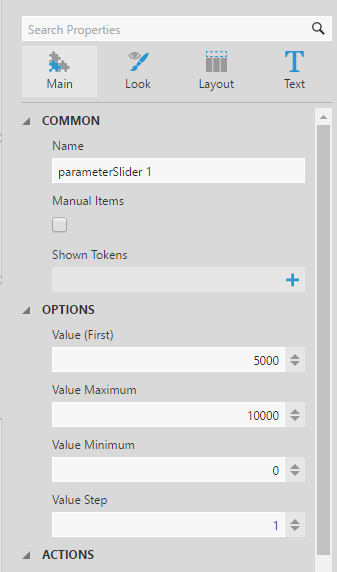Change the properties for the slider filter

You and other viewers can now change the value used in this formula.

## 8. Filtering

When filtering a metric set that contains formula measures, formulas are calculated after filtering is applied, except for filtering by formula measure values. These operations occur in this order:

1. The metric set's data is filtered by hierarchy values and by measures that are not formulas
2. Formula functions run their calculations based on this data
3. The resulting data is then filtered by formula measure values

For example, the following table shows a formula measure using the Percent of Total function, which was calculated based on the entire set of rows. Filtering this formula measure to a range of 0.15 (15%) and up preserves and filters by these calculations, displaying only one row: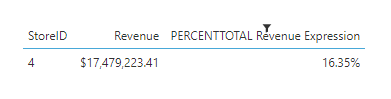Filtering by a PERCENTTOTAL formula measure

Tip
When you need formulas to be calculated before some filtering is applied, add a formula measure to filter by instead. This formula can compare against hierarchy values or a parameter's value.

## 9. Notes

• When formulas are calculated for the members of a time dimension hierarchy at the Week level, and the results are visualized in a chart set to use a Date/Time scale, the Week 53 member of one year and Week 1 member of the following year often must be combined together into a single member representing the full seven days. In this case, the aggregator set on the formula measure is used to combine the two formula results together in most cases such as for aggregators Sum, Average, Minimum, Maximum, and Count. For other aggregators, one of the two week's values may be displayed.